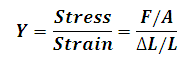# Young's Modulus Calculator

This Calctown Calculator calculates the young's modulus of a body under a certain stress.

N
m2
m
m

#### Result

N/m2
N/m2where

Y = young's modulus

F = force exerted on an object under tension

A = cross-sectional area through which the force is applied

ΔL = amount by which the length of the object changes

L = original length of the object.